Wednesday, August 31, 2011

CS106A Programming Methodology Problem Solutions: Assignment 2

EDIT: Source code files now available for download!

Assignment two has a total of six problems, and the problems are considerably harder than those in assignment one. This is partly because of the introduction of the graphics program, and partly because of the introduction of return methods (for it creates a lot more possibilities for program decomposition). On the bright side, in this assignment we are allowed to use variables as well as various keywords (e.g., "break"), making it a lot easier to program.

Anyway, without further ado, I'll get to the problems:

For this problem, you have to ask the user for the coefficients of a quadratic equation. Then, using the quadratic formula, your program should output the solutions. Or, if the equation has no solution, then your program should output a line saying so.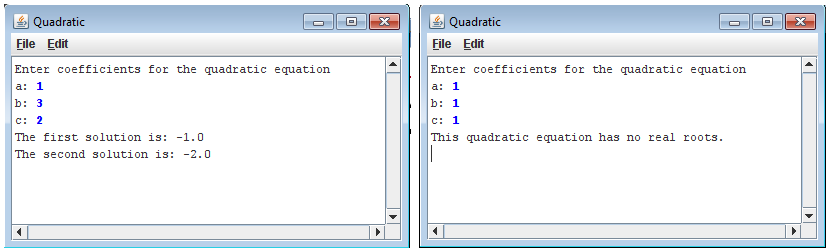click to enlarge

This problem is very straight-forward.
// --------------------
//This program is a stub for the Quadratic problem, which finds the
// roots of the quadratic equation.

import acm.program.*;

public class Quadratic extends ConsoleProgram {

private void userInput() {
//Get user input:
println("Enter coefficients for the quadratic equation ");
}

private void printSolutions() {
int  discriminant=b*b-4*a*c; //calculates number inside the root
double firstSolution, secondSolution;

//Display appropriate messages based on whether there are solutions
if (discriminant<0){
println("This quadratic equation has no real roots.");
} else {
//Calculate solutions
firstSolution=(-b+Math.sqrt(discriminant))/(2*a);
secondSolution=(-b-Math.sqrt(discriminant))/(2*a);
//Output solutions
println("The first solution is: "+firstSolution);
println("The second solution is: "+secondSolution);
}
}
public void run() {
userInput (); //get the coefficients
printSolutions(); //output message
}

//Private instance variables
private static int a,b,c;

}

Problem 2: Find Range (FindRange.java)
This program reads in a list of integers, one per line, until a sentinel value of 0 (which could be easily changed to some other value) is entered. Then, the program would output the largest and smallest integers from the list, excluding the sentinel. If the very first line of input contains the sentinel value, then the program should indicate so. And if the second line of input contains the sentinel value, the program should output the first integer as both the largest and the smallest integer.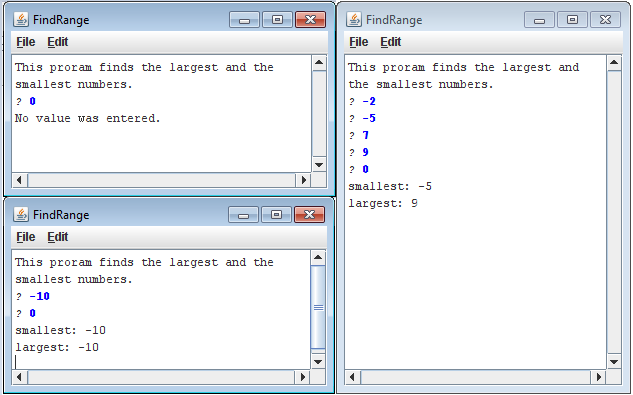click to enlarge

Although the program itself is simple, it is quite easy to make some logic errors. In fact, I had to fix my code a couple of times, for it failed a few certain cases.

//File: FindRange.java
//--------------------
//This program is a stub for the FindRange problem, which finds the
//smallest and largest values in a list of integers.

import acm.program.*;

public class FindRange extends ConsoleProgram {

private static final int SENTINEL=0;

//Intro: description of program
private void intro () {
println ("This proram finds the largest and the smallest numbers.");
}

//Gets user input until sentinel is entered
private void userInput () {
int input;
while (true) {

//Exits when sentinel is entered
if (input==SENTINEL) {
break;
}

//Update values of maxNum and minNum if appropriate
if (input>maxNum||maxNum==SENTINEL) {
maxNum=input;
}

if (minNum>input||minNum==SENTINEL) {
minNum=input;
}

}
}

private void printResults() {

//If the first input is the sentinel, then the boolean expression will
//be false and no values will be output. If not, then the largest and
//smallest numbers will be output
if (minNum!=SENTINEL) {
println ("smallest: "+minNum);
println ("largest: "+maxNum);

} else {
println ("No value was entered.");
}

}
public void run() {
intro(); //outputs program description
userInput(); //gets a list of numbers from user
printResults(); //Outputs the largest and smallest nums
}

//Private instance variables:
private static int maxNum=SENTINEL, minNum=SENTINEL;
}

Problem 3: Hailstone (Hailstone.java)
This program is extremely simple. You first take an integer from the user, then,
• if the number is odd, multiply the integer by 3, then add one to the product
• if the number is even, divide it by two
You then repeat this process until the resulting number is 1. At this point, the program will output the number of steps that the process took.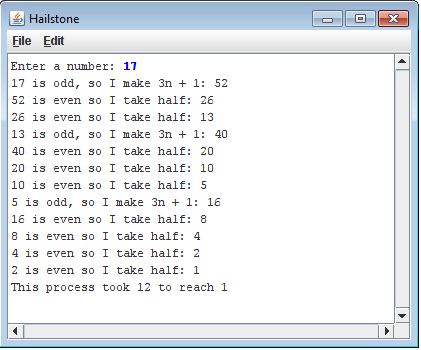// File: Hailstone.java
//--------------------
//This program is a stub for the Hailstone problem, which computes
//Hailstone sequence described in Assignment #2.

//A while loop will do...

import acm.program.*;

public class Hailstone extends ConsoleProgram {

public void run() {
int num;
int count=0 ;//The number of steps it takes
num=readInt("Enter a number: ");

while (num!=1) {
if (num%2==0) {
print (num+" is even so I take half: ");
num=num/2;
} else {
print (num+" is odd, so I make 3n + 1: ");
num=num*3+1;
}
println(num);
count++;

}
println("This process took "+ count+ " to reach 1");
}
}

Problem 4: Target (Target.java)
For this program, you basically have to draw three filled ovals centered in the screen:// File: Target.java
// ------------------
//This program is a stub for the Target problem, which displays
//a target by adding concentric circles to the canvas.

//The only thing problem is that the final size might be off by one pixel because
//of rounding

import java.awt.Color;
import acm.graphics.*;
import acm.program.GraphicsProgram;

public class Target extends GraphicsProgram {

private static final double INCHES_1=1, INCHES_2=0.65, INCHES_3=0.3;// the three radii

public void run() {
//Specify the radius (in inches) and color for each circle:
}

//This method adds a filled circle (with specified color and size) to the center of the canvas
private void addFilledCricleToCenter (double radiusInInches, Color col) {
int diameter= radiusInPixels*2; //calculate diameter
int startX= (getWidth()/2)- radiusInPixels;  //The leftmost x-location of the circle
int startY= (getHeight()/2)- radiusInPixels; //the topmost y-location of the circle

//create filled circle with the appropriate attributes, and add it to the canvas:
add(fillOval(startX, startY, diameter, diameter, col));

}

private int convertToPixels (double inches){
return (int)(Math.round(inches*72));
}

//This method creates a filled oval with the appropriate color, location and size
private GOval fillOval (int x, int y, int width, int height, Color col) {
GOval oval= new GOval(x, y, width, height); //Create oval with the appropriate size and loc.
oval.setColor(col); //Change colour
oval.setFillColor (col); //change colour
oval.setFilled(true); //make it a filled circle
return oval;
}
}

Problem 5: GraphicsHierarchy (GraphicsHierarchy.java)
This program should output something similar to the image below: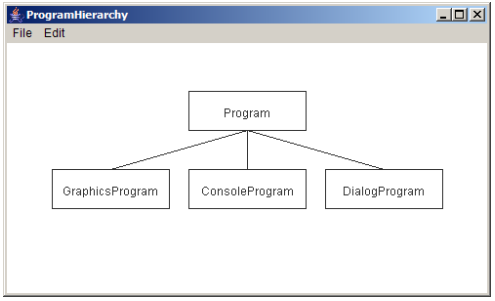The diagram should always be centered in the screen regardless of the width and height of the boxes. Also, the texts should be centered in the boxes. This was probably the program that I worked the longest on, for I am pretty slow at centering objects. It also took me quite a while to simplify the code and make it more general. But besides that, there wasn't any complicated logic needed to code this program.
//
// File: GraphicsHierarchy.java
//----------------------------
//This program is a stub for the GraphicsHierarchy problem, which
//draws a partial diagram of the acm.graphics hierarchy.

import acm.program.*;
import acm.graphics.*;

public class GraphicsHierarchy extends GraphicsProgram {

private static final int BOX_HEIGHT=50, BOX_WIDTH=200;
private static final int VERT_DISTANCE=40, HORI_DISTANCE=50; // The horizontal and vertical distance between any two adjacent boxes

public void run() {
assignValues(); //Assign values to the variables that hold the coordinates of the boxes

drawBoxes();
drawLines();

}

private void assignValues() {
//the console window will only be created after the run method is called, and before that, the height and width will both be
//zero. Therefore, to get the following three variables to have proper values, the values must be assigned in a method.
topBoxY=getHeight()/2-(BOX_HEIGHT*2+VERT_DISTANCE)/2;
bottomBoxY=getHeight()/2+ VERT_DISTANCE/2;
centerBoxX=(getWidth()-BOX_WIDTH)/2;
}

//Add the labels. StartX and startY are the coordinates of the top-left corner of the rectangle that they're in
private void addLabel(String labelText, int startX, int startY) {
GLabel lab= new GLabel (labelText, startX, startY); //Create label
lab.move ((BOX_WIDTH-lab.getWidth())/2,(BOX_HEIGHT+lab.getAscent())/2 ); //move to center
}

//Draw the lines
private void drawLines() {
//All three lines branch out of one point, and startX and startY are the coordinates of that point
int startX=getWidth()/2;
int startY=(int)((getHeight()-VERT_DISTANCE)/2);

//Draw the three lines
for(int i=-1; i<=1; i++) {
add(new GLine(startX, startY, startX+i*(BOX_WIDTH+HORI_DISTANCE), startY+VERT_DISTANCE));
}

}

//Draws the four boxes
private void drawBoxes () {
//The box at the top:
add(new GRect(centerBoxX, topBoxY, BOX_WIDTH, BOX_HEIGHT));

//The boxes at the bottom
for (int i=-1; i<=1; i++) {
add(new GRect(centerBoxX+(BOX_WIDTH+HORI_DISTANCE)*i, bottomBoxY, BOX_WIDTH, BOX_HEIGHT));
}
}

//Private instance variables:
//These are the variables that hold the coordinates of the boxes. See "assignValues" method for more info.
private static int topBoxY;
private static int bottomBoxY;
private static int centerBoxX;
}

Problem 6: Pyramid (Pyramid.java)
With the specified brick width, brick height, and number of rows, the program should output a pyramid like the one below, centered in the screen.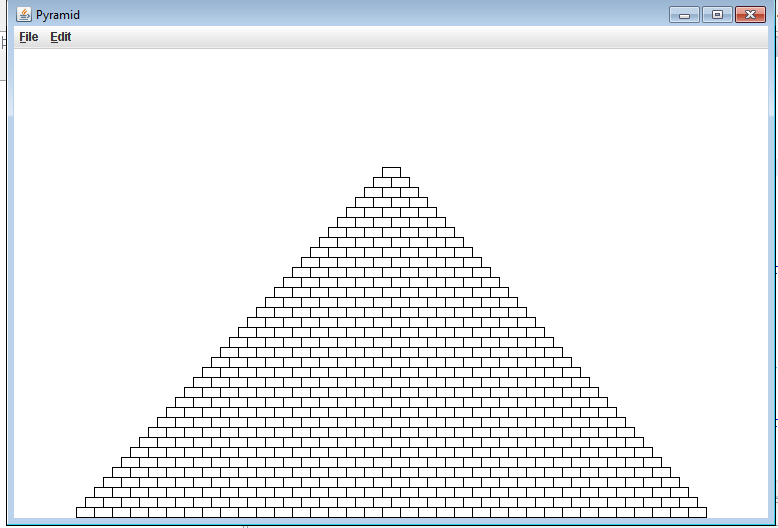// File: Pyramid.java
// ------------------
// This program is a stub for the Pyramid problem, which draws
// a brick pyramid.

import acm.graphics.*;
import acm.program.*;

public class Pyramid extends GraphicsProgram {

private static int BRICK_WIDTH=18;
private static int BRICK_HEIGHT=10;
private static int BRICKS_IN_BASE=35;

public void run() {

//Draw the rectangles row by row, from the bottom row to the top row
for (int i=BRICKS_IN_BASE; i>=1; i--) {
int yLocation=getHeight()-(BRICKS_IN_BASE-i+1)*BRICK_HEIGHT-1;
fillRow (yLocation, i);
}
}

private void fillRow (int yLocation, int numOfBricks) {
int startX=(getWidth()- numOfBricks*BRICK_WIDTH)/2; //the x-Location of the leftmost brick
//Use for loop to draw the bricks
//!!!Code continued at the bottom because of the syntaxHighlighter's glitch!
This is the rest of the code:
for (int i=0; i<numOfBricks; i++) { add(new GRect(startX+i*BRICK_WIDTH, yLocation, BRICK_WIDTH, BRICK_HEIGHT)); } } }